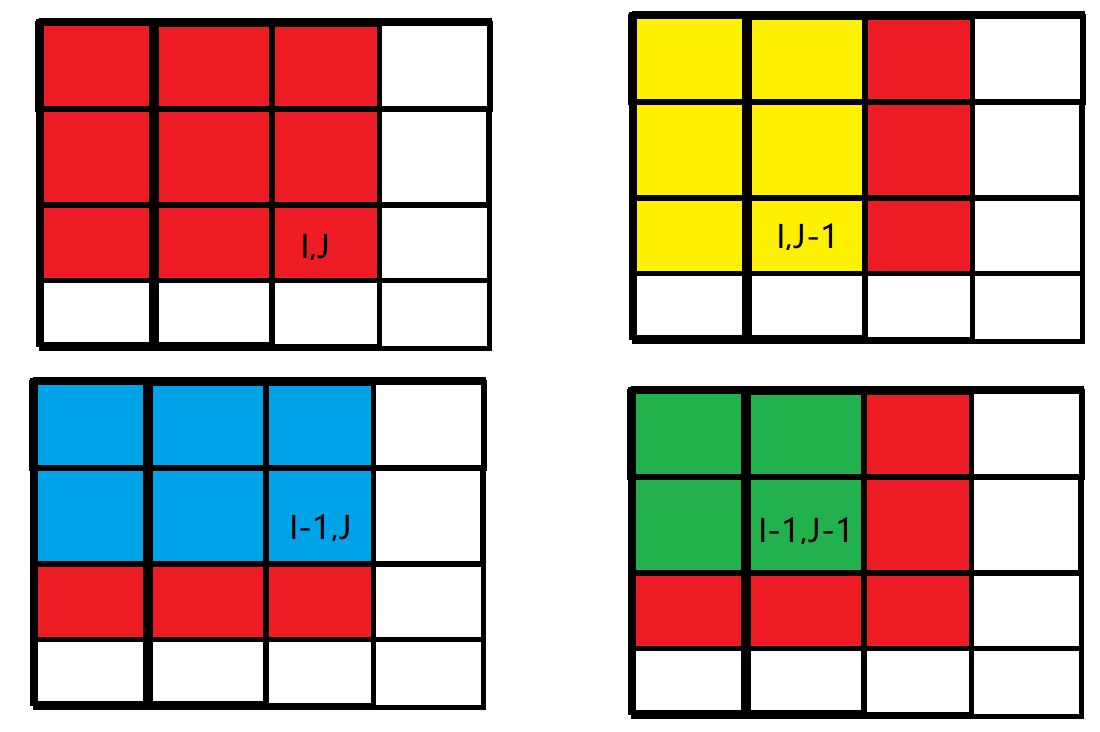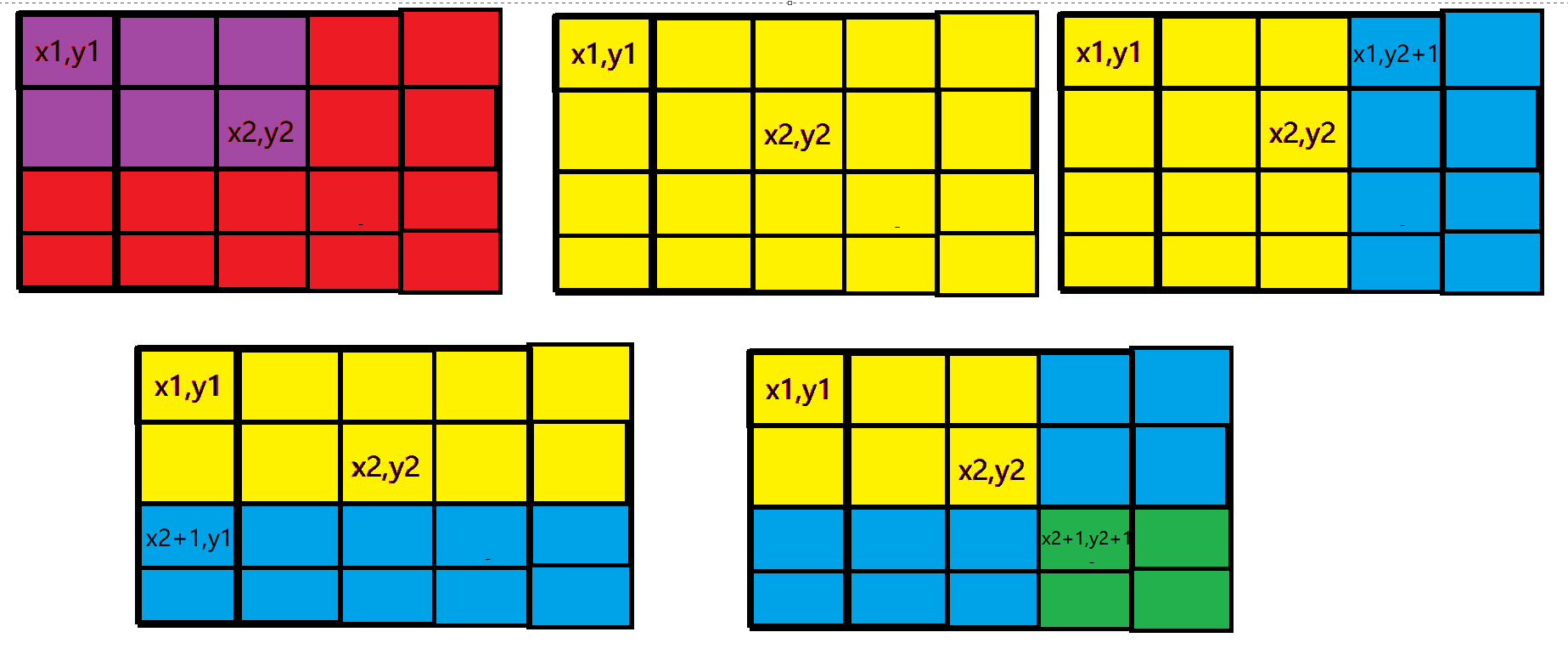• ## 差分矩阵

千次阅读 2019-11-09 16:22:20
思想 其实就是把一维的差分数组变成二维的了，只要搞清楚递推公式就行，b[i][j]=a[i][j]-a[i][j-1]-a[i-1][j]+a[i-1][j-1]；...最重要的求差分的公式： b[x1][y1] += c; b[x2+1][y2+1] += c; b[x1][y2 + 1] -...
思想
其实就是把一维的差分数组变成二维的了，只要搞清楚递推公式就行，b[i][j]=a[i][j]-a[i][j-1]-a[i-1][j]+a[i-1][j-1]；最后求前缀和的公式b[i][j] += b[i][j - 1]+b[i-1][j]-b[i-1][j-1]; 最重要的求差分的公式： b[x1][y1] += c; b[x2+1][y2+1] += c; b[x1][y2 + 1] -= c; b[x2 + 1][y1] -= c;
不懂得画一下图就清楚了
代码
#include <stdio.h>
#include <algorithm>
#include <iostream>
#include <iomanip>
#include <vector>
using namespace std;

int b;
int a;
int main() {
int n, m,k;
cin >> n >> m >>k;
for (int i = 1; i <= n; i++) {
for (int j = 1; j <= m; j++) {
scanf_s("%d", &a[i][j]);
b[i][j] = a[i][j] - a[i][j - 1] - a[i - 1][j] + a[i - 1][j - 1];
}
}
for (int i = 1; i <= k; i++) {
int x1,x2,y1,y2,c;
cin >> x1 >> y1 >> x2 >> y2 >> c;
b[x1][y1] += c;
b[x2+1][y2+1] += c;
b[x1][y2 + 1] -= c;
b[x2 + 1][y1] -= c;
}
for (int i = 1; i <= n; i++) {
for (int j = 1; j <= m; j++) {
b[i][j] += b[i][j - 1]+b[i-1][j]-b[i-1][j-1];
cout << b[i][j] << " ";
}
cout << "\n";
}
}

展开全文• 通过定义的基元扫描图像，生成像素差分矩阵以及基元过渡矩阵。最后利用统计算法将上述两个矩阵合并为一个像素差分基元矩阵，实现了颜色、纹理以及空间信息等多特征的图像检索。在Corel标准图像数据库中执行图像检索...图像检索
• k阶差分方程以矩阵方式给出，形如 A = [a0, a1, a2 ,... ak-1]  [ 1, 0, 0,... 0]  [0, 1, 0,... 0] ......  [0, 0, 0.. 1, 0] 朴素的求解这个矩阵的n次幂，bn = A*b0，时间复杂度高达O(k^3 LogN)
k阶差分方程以矩阵方式给出，形如
A =  [a0, a1, a2 ,... ak-1]
[ 1,    0,     0,...       0]
[0,     1,    0,...       0]
......
[0, 0,0.. 1,   0]
朴素的求解这个矩阵的n次幂，bn = A*b0，时间复杂度高达O(k^3 LogN)。
下面，将优化差分方程的时间复杂度为O(K^2 LogN)。
Cayley Hamilton定理:
设$A$ 为给定的$n \times n$ 矩阵，并设$I_n$ 为$n \times n$ 单位矩阵，则$A$ 的特征多项式定义为：$p(\lambda)=\det(\lambda I_n-A)\,$
其中 det 表行列式函数。凯莱-哈密顿定理断言：$p(A)=0.\,$ (wiki)
反复利用上述关系，我们就能将A^k用A^0..A^(n-1)线性表示，且仅仅需要进行多项式的运算，时间复杂度大大降低。
bn = b0*(c_0*A^0 + c_1*A^1 +...+c_(k-1) * A^(k-1) ) = c_0 * b0 + c_1 * b1 + ... + c_(k-1) * b_(k-1)
因此，我们只需要预处理出b0..b_(2n-1)，利用多项式乘法和快速幂得到A^n关于A^0..A^(k-1)的系数，就能求得bn，时间复杂度为多项式相乘+展开，总的复杂度为O(K^2logN)。

leslie矩阵形式与矩阵M类似，得到特征多项式之后，只需要做多项式乘法，最后有初值条件得到人口分布既可。

对于非齐次线性差分方程，仅仅是特征多项式不同，思路类似。


展开全文• ## 差分——（2）二维差分

千次阅读 多人点赞 2020-02-25 23:28:30
下面我们扩展一下，来介绍二维差分。 什么是二维差分 我们有一个矩阵，如下图所示。 根据二维前缀和表示的是右上角矩形的和，由于差分只涉及前面相邻的数（由一维可以推出），并且由前面范围的数相加得到这个...
前面部分我们介绍了一维差分，https://blog.csdn.net/justidle/article/details/103761632。下面我们扩展一下，来介绍二维差分。
什么是二维差分
我们有一个矩阵，如下图所示。根据二维前缀和表示的是右上角矩形的和，由于差分只涉及前面相邻的数（由一维可以推出），并且由前面范围的数相加得到这个位置的数。那么类比二维前缀和和一维差分，可以简单推测出二维差分的公式$p(A)=0.\,$
如何从差分矩阵得到原矩阵呢？可以参考下面公式$p(A)=0.\,$
P.S. 道歉，前面这个公式写错了，感谢 @繁星-落眼 的纠正。再次道歉。
举例
比如，我们有一个矩阵 a，如下所示：
1 2 4 3
5 1 2 4
6 3 5 9
那么对应的二维差分矩阵 p 如下：
1  1  2 -1
4 -5 -1  3
1  1  1  2
应用
如果我们要在左上角是 (x1,y1)，右下角是 (x2,y2) 的矩形区间每个值都 +a，如下图所示在我们要的区间开始位置（x1,y1）处 +c，根据前缀和的性质，那么它影响的就是整个黄色部分，多影响了两个蓝色部分，所以在两个蓝色部分 -c 消除 +c 的影响，而两个蓝色部分重叠的绿色部分多了个 -c 的影响，所以绿色部分 +c 消除影响。所以对应的计算方法如下：
diff[x1][y1] += c;
diff[x1][y2+1] -=c;
diff[x2+1][y1] -=c;
diff[x2+1][y2+1] += c;

模板题
链接
我的OJ，http://47.110.135.197/problem.php?id=5227。
题目描述
输入一个 n 行 m 列的整数矩阵，再输入 q 个操作，每个操作包含五个整数 x1，y1，x2，y2，c，其中 (x1, y1) 和 (x2, y2) 表示一个子矩阵的左上角坐标和右下角坐标。 每个操作都要将选中的子矩阵中的每个元素的值加上 c。 请你将进行完所有操作后的矩阵输出。
输入
第一行包含整数 n，m，q。 接下来 n 行，每行包含 m 个整数，表示整数矩阵。 接下来 q 行，每行包含 5 个整数 x1，y1，x2，y2，c，表示一个操作。
输出
共 n 行，每行 m 个整数，表示所有操作进行完毕后的最终矩阵。
样例输入
3 4 3
1 2 2 1
3 2 2 1
1 1 1 1
1 1 2 2 1
1 3 2 3 2
3 1 3 4 1
样例输出
2 3 4 1
4 3 4 1
2 2 2 2
数据范围
1 ≤ n, m ≤ 1000, 1 ≤ q ≤ 100000, 1 ≤ x1 ≤ x2 ≤ n, 1 ≤ y1 ≤ y2 ≤ m, −1000 ≤ c ≤ 1000, −1000 ≤ 矩阵内元素的值 ≤ 1000
分析
这是一个二维差分的模板题。
数据分析
下面我们根据样例输入来分析一下，样例输出是如何得到的。
初始状态的差分数组 diff 为
 1  1 0 -1
2 -2 0  0
-2  1 0  1
第一次操作为 1 1 2 2 1，得到差分数组 diff 变为
 2  1 -1 -1
2 -2  0  0
-3  1  1  1
第二次操作为 1 3 2 3 2，得到差分数组 diff 变为
 2  1  1 -3
2 -2  0  0
-3  1 -1  3
第二次操作为 1 3 2 3 2，得到差分数组 diff 变为
 2  1  1 -3
2 -2  0  0
-2  1 -1  3
最终，我们可以根据差分数组 diff 求出对应的数组。
数据范围
从题目中知道，n 的最大值为 1000，因此我们定义数组为 1004。
数组的每个数范围为 [-1000, 1000]，c 的范围为 [-1000, 1000]，操作数 q 最大值为 100000。因此我们可以计算出，经过 q 次操作后，最大的数据为 1000+1000*100000 = 10^8+1000，在 int 的表示范围内。同理最小的数据将是 -1000+(-1000*100000)=-10^8-1000，也在 int 的表示范围内。
AC 代码
#include <bits/stdc++.h>
using namespace std;

const int MAXN = 1e3+6;
const int MAXM = 1e3+6;
int a[MAXN][MAXM] = {};
int diff[MAXN][MAXM] = {};

int main() {
int n,m,q;
scanf("%d%d%d", &n, &m, &q);

int i, j;
for (i=1; i<=n; i++) {
for (j=1; j<=m; j++) {
scanf("%d", &a[i][j]);
diff[i][j] = a[i][j]-a[i-1][j]-a[i][j-1]+a[i-1][j-1];
}
}

for (i=0; i<q; i++) {
int x1, y1, x2, y2, c;
scanf("%d%d%d%d%d", &x1, &y1, &x2, &y2, &c);
diff[x1][y1] += c;
diff[x1][y2+1] -=c;
diff[x2+1][y1] -=c;
diff[x2+1][y2+1] += c;
}

for (i=1; i<=n; i++) {
for (j=1; j<=m; j++) {
diff[i][j] += diff[i-1][j]+diff[i][j-1]-diff[i-1][j-1];
printf("%d ", diff[i][j]);
}
printf("\n");
}

return 0;
}

展开全文• 协方差矩阵定义及其计算公式   (2014-03-20 18:36:56) 转载▼   分类： matlab 协方差矩阵在机器学习中经常用到，查看wiki：http://zh.wikipedia.org/wiki/协方差矩阵 可知...

协方差矩阵的定义及其计算公式(2014-03-20 18:36:56)转载▼

分类： matlab

协方差矩阵在机器学习中经常用到，查看wiki：http://zh.wikipedia.org/wiki/协方差矩阵 可知协方差矩阵的具体计算公式如下：
在统计学与概率论中，协方差矩阵是一个矩阵，其每个元素是各个向量元素之间的协方差。这是从标量随机变量到高维度随机向量的自然推广。
假设是以个标量随机变量组成的列向量，并且是其第i个元素的期望值，即,。协方差矩阵被定义的第i，j项是如下：即：矩阵中的第个元素是与的协方差。这个概念是对于标量随机变量方差的一般化推广。

二、理解的关键
1、理解的关键是两个随机变量x1,x2的协方差如何计算，有cov(x1,x2) = E{[(x1-E(x1)][x2-E(x2)]}，对于离散的随机变量x1,x2,协方差矩阵描述的x1,x2相互联系的偏差，所以两个随机变量是一一对应的，即假设有m个样本值，则分别为(x11,x21),(x12,x22),(x13,x23),...(x1m,x2m)，这便可以写成一个2*m的矩阵的形式。则x1,x2协方差表示的是两个随机变量对应的样本值分别都减去各自均值后的乘积的均值（因为无偏性估计的缘故，除以的不是m而是m-1)；
2、所以对于一个n*m的样本矩阵，得出的协方差矩阵C是n*n的矩阵，协方差矩阵每个元素Cij表示的随机变量xi,xj的协方差。所以协方差矩阵是一个对称矩阵，且对角线上元素为每个随机变量的方差（如果是信号的话可以看成是能量）；如果各个变量相关性很小的话，互相的协方差接近0，即协方差矩阵基本上为对角阵；
3、可以证明，协方差矩阵是非负定矩阵，这可以有非负定矩阵的定义得到；
4、同样地，为了表示各个随机变量相关性到底有多大，可以引入相关性矩阵。
统计学的基本概念
学过概率统计的孩子都知道，统计里最基本的概念就是样本的均值，方差，或者再加个标准差。首先我们给你一个含有n个样本的集合，依次给出这些概念的公式描述，这些高中学过数学的孩子都应该知道吧，一带而过。
均值：标准差：方差：很显然，均值描述的是样本集合的中间点，它告诉我们的信息是很有限的，而标准差给我们描述的则是样本集合的各个样本点到均值的距离之平均。以这两个集合为例，[0，8，12，20]和[8，9，11，12]，两个集合的均值都是10，但显然两个集合差别是很大的，计算两者的标准差，前者是8.3，后者是1.8，显然后者较为集中，故其标准差小一些，标准差描述的就是这种“散布度”。之所以除以n-1而不是除以n，是因为这样能使我们以较小的样本集更好的逼近总体的标准差，即统计上所谓的“无偏估计”。而方差则仅仅是标准差的平方。
为什么需要协方差？
上面几个统计量看似已经描述的差不多了，但我们应该注意到，标准差和方差一般是用来描述一维数据的，但现实生活我们常常遇到含有多维数据的数据集，最简单的大家上学时免不了要统计多个学科的考试成绩。面对这样的数据集，我们当然可以按照每一维独立的计算其方差，但是通常我们还想了解更多，比如，一个男孩子的猥琐程度跟他受女孩子欢迎程度是否存在一些联系啊，协方差就是这样一种用来度量两个随机变量关系的统计量，我们可以仿照方差的定义：来度量各个维度偏离其均值的程度，标准差可以这么来定义：协方差的结果有什么意义呢？如果结果为正值，则说明两者是正相关的(从协方差可以引出“相关系数”的定义)，也就是说一个人越猥琐就越受女孩子欢迎，那必须的~结果为负值就说明负相关的，越猥琐女孩子越讨厌，可能吗？如果为0，也是就是统计上说的“相互独立”。
从协方差的定义上我们也可以看出一些显而易见的性质，如：协方差多了就是协方差矩阵
上一节提到的猥琐和受欢迎的问题是典型二维问题，而协方差也只能处理二维问题，那维数多了自然就需要计算多个协方差，比如n维的数据集就需要计算个协方差，那自然而然的我们会想到使用矩阵来组织这些数据。给出协方差矩阵的定义：这个定义还是很容易理解的，我们可以举一个简单的三维的例子，假设数据集有三个维度，则协方差矩阵为可见，协方差矩阵是一个对称的矩阵，而且对角线是各个维度上的方差。
Matlab协方差实战
上面涉及的内容都比较容易，协方差矩阵似乎也很简单，但实战起来就很容易让人迷茫了。必须要明确一点，协方差矩阵计算的是不同维度之间的协方差，而不是不同样本之间的。这个我将结合下面的例子说明，以下的演示将使用Matlab，为了说明计算原理，不直接调用Matlab的cov函数(蓝色部分为Matlab代码)。
首先，随机产生一个10*3维的整数矩阵作为样本集，10为样本的个数，3为样本的维数。
1MySample = fix(rand(10,3)*50)根据公式，计算协方差需要计算均值，那是按行计算均值还是按列呢，我一开始就老是困扰这个问题。前面我们也特别强调了，协方差矩阵是计算不同维度间的协方差，要时刻牢记这一点。样本矩阵的每行是一个样本，每列为一个维度，所以我们要按列计算均值。为了描述方便，我们先将三个维度的数据分别赋值：
dim1 = MySample(:,1);dim2 = MySample(:,2);dim3 = MySample(:,3);
计算dim1与dim2，dim1与dim3，dim2与dim3的协方差：
1sum( (dim1-mean(dim1)) .* (dim2-mean(dim2)) ) / ( size(MySample,1)-1 ) %(10行；（10-1）) 得到 74.53332；sum( (dim1-mean(dim1)) .* (dim3-mean(dim3)) ) / ( size(MySample,1)-1 ) % 得到  -10.08893；sum( (dim2-mean(dim2)) .* (dim3-mean(dim3)) ) / ( size(MySample,1)-1 ) % 得到  -106.4000
搞清楚了这个后面就容易多了，协方差矩阵的对角线就是各个维度上的方差，下面我们依次计算：
1std(dim1)^2 % 得到   108.3222；2std(dim2)^2 % 得到   260.6222；3std(dim3)^2 % 得到   94.1778
这样，我们就得到了计算协方差矩阵所需要的所有数据，调用Matlab自带的cov函数进行验证：
1cov(MySample)把我们计算的数据对号入座，是不是一摸一样？
Update：今天突然发现，原来协方差矩阵还可以这样计算，先让样本矩阵中心化，即每一维度减去该维度的均值，使每一维度上的均值为0，然后直接用新的到的样本矩阵乘上它的转置，然后除以(N-1)即可。其实这种方法也是由前面的公式通道而来，只不过理解起来不是很直观，但在抽象的公式推导时还是很常用的！同样给出Matlab代码实现：
1X = MySample - repmat(mean(MySample),10,1);    % 中心化样本矩阵，使各维度均值为02C = (X'*X)./(size(X,1)-1);%B = repmat(A,m,n)将矩阵 A 复制 m×n 块，即把 A 作为 B 的元素，B 由 m×n 个 A 平铺而成。B 的维数是 [size(A,1)*m, (size(A,2)*n]

>> A = [1,2;3,4]

A =

1 2

3 4

>> B = repmat(A,2,3)

B =

1 2
1 2
1 2

3 4
3 4
3 4

1 2
1 2 1 2

3 4
3 4 3 4

总结
理解协方差矩阵的关键就在于牢记它计算的是不同维度之间的协方差，而不是不同样本之间，拿到一个样本矩阵，我们最先要明确的就是一行是一个样本还是一个维度。
matlab：
1、均值 数学定义： Matlab 函数：mean >>X=[1,2,3] >>mean(X)=2 如果X 是一个矩阵，则其均值是一个向量组。mean(X,1)为列向量的均值，mean(X,2)为行向量的均 值。 >>X=[1 2 3          4 5 6] >>mean(X,1)=[2.5, 3.5, 4.5] >>mean(X,2)=[2                        5] 若要求整个矩阵元素的均值，则为mean(mean(X))。 >>mean(mean(X))=3.5 也可使用mean2函数： >>mean2(X)=3.5 median，求一组数据的中值，用法与mean 相同。 >>X=[1,2,9] >>mean(X)=4 >>median(X)=2 2、方差 数学定义： 均方差： Matlab 函数：var 要注意的是var 函数所采用公式中，分母不是，而是。这是因为var 函数实际上求的并不是方差， 而是误差理论中“有限次测量数据的标准偏差的估计值”。 >>X=[1,2,3,4] >>var(X)=1.6667 >> sum((X(1,:)-mean(X)).^2)/length(X)=1.2500 >> sum((X(1,:)-mean(X)).^2)/(length(X)-1)=1.6667 var 没有求矩阵的方差功能，可使用std 先求均方差，再平方得到方差。 std，均方差，std(X,0,1)求列向量方差，std(X,0,2)求行向量方差。 >>X=[1 2          3 4] >>std(X,0,1)=1.4142 1.4142 >>std(X,0,2)=0.7071                      0.7071 若要求整个矩阵所有元素的均方差，则要使用std2函数： >>std2(X)=1.2910 4、协方差矩阵 A=[61.45,55.9,61.95,59,58.14,53.61,55.48,54.21,61.52,54.92]; B=[40.36,39.8,49.2,48,51.5,49.39,51.13,58.06,61,62.35]; C=[8.61,8.91,10.43,13.32,13.48,15.75,18.14,19.95,21.95,23.53]; D=[14.31,14.72,15.28,15.91,14.67,15,15.86,15.16,13.72,12.94]; E=[7.67,7.75,8.15,9.24,10.68,10.58,10.31,10,8.91,8.51]; >> q=[A',B',C',D',E']; >> w=cov(q) w = 10.3710 -4.7446 -6.6023 -0.1873 -1.8881 -4.7446 59.1503 38.7606 -3.0743 3.0982 -6.6023 38.7606 28.6966 -2.0199 2.4166 -0.1873 -3.0743 -2.0199 0.8474 0.3936
-1.8881 3.0982 2.4166 0.3936 1.3412
例：
a =     -1     1     2     -2     3     1      4     0     3  for i=1:size(a,2) %size(a,1)求矩阵的行数；size(a,2)求矩阵的列数，相当于length(a)；size(a)同时求矩阵的行和列数     for j=1:size(a,2) %A(1,:)表示这个矩阵的第一行；A(:,1)表示这个矩阵的第一列
c(i,j)=sum((a(:,i)-mean(a(:,i))).*(a(:,j)-mean(a(:,j))))/(size(a,1)-1);     end  end
c =
10.3333   -4.1667    3.0000
-4.1667    2.3333   -1.5000
3.0000   -1.5000    1.0000
c为求得的协方差矩阵，在matlab以矩阵a的每一列为变量，对应的每一行为样本。这样在矩阵a中就有3个列变量分别为a（:,1), a(:,2), a(:,3)。
在协方差矩阵c中，每一个元素c(i,j)为对第i列与第j列的协方差，例如c(1,2) = -4.1667为第一列与第二列的协方差。

拿c(1,2)的求解过程来说
c(1,2)=sum((a(:,1)-mean(a(:,1))).*(a(:,2)-mean(a(:,2))))/(size(a,1)-1);
1. a(:,1)-mean(a(:,1))，第一列的元素减去该列的均值得到
-1.3333
-2.3333
3.6667
2,  a(:,2)-mean(a(:,2))，第二列的元素减去该列的均值得到
-0.3333
1.6667
-1.3333
3, 再将第一步与第二部的结果相乘
-1.3333         -0.3333          0.4444
-2.3333  .*     1.6667  =        -3.8889
3.6667          -1.3333          -4.8889

4, 再将结果求和/size(a,1)-1 得 -4.1667,该值即为c（1,2）的值。
再细看一下是不是与协方差公式：Cov(X,Y) = E{ [ (X-E(X) ] [ (Y-E(Y) ] } 过程基本一致呢，只是在第4步的时候matlab做了稍微的调整，自由度为n-1，减少了一行的样本值个数。
clear all; a =[-1,1,2;-2,3,1;4,0,3] b=cov(a) for i=1:size(a,2) %size(a,1)求矩阵的行数；size(a,2)求矩阵的列数     for j=1:size(a,2) %A(1,:)表示这个矩阵的第一行；A(:,1)表示这个矩阵的第一列     c(i,j)=sum((a(:,i)-mean(a(:,i))).*(a(:,j)-mean(a(:,j))))/(size(a,1)-1)     end end

展开全文• 本文介绍了图像特征分析的常用方法，包括颜色矩、颜色直方图、灰度差分统计、自相关函数、灰度共生矩阵。介绍了这些特征量的基本概念、特征规律、以及通过Matlab的实现方法与代码。计算机视觉 matlab 机器学习 数字图像处理
• f（x，y）对X求导，则 =0列向量=D'X+H（X）*X X=-D'X/H（X） ///////////////////////顺便对比一下协方差矩阵： 协方差矩阵定义：C=c11 c12 =σ1^2 ρ*σ1*σ2 c21 c22 ρ*σ1*σ2 σ2^2 矩阵A= σ2^2 -ρ*σ...
• 定义 设函数y = f(x)在x.的邻域内有定义，x0及x0 + Δx在此区间内。如果函数的增量Δy = f(x0 + Δx) −f(x0)可表示为 Δy = AΔx0 +o(Δx0)（其中A是不依赖于Δx的常数），而o(Δx0)是比Δx高阶的无穷小，那么称...工具
• MATLAB的基本知识点： 1. 变量的定义以及特殊标量的表示与含义 2. 行向量的定义与赋值 3. 矩阵定义与赋值以及特殊矩阵的生成 每一个都有实例，并且都上机实践过的，能让为你打下很好的学习MATLAB编程的基础。算法 MATLAB
• 纹理特征四：NGTDM–邻域灰度差矩阵 1. NGTDM 的解释与计算 定义： 假设i=f(k,l)i=f(k,l)i=f(k,l)是坐标为(k,l)(k,l)(k,l)上的像素值。那么点(k,l)(k,l)(k,l)的周围的平均灰度值Ai‾\overline{A_i}Ai​​的计算...NGTDM 纹理特征 图像处理
• 根据T函数自身输入与输出结构特点,结合T函数窄度相关定义,研究了T函数线性性质和差分性质。通过构造马尔可夫链和概率转移矩阵,给出了其Walsh谱值与差分转移概率计算的多项式时间快速算法,时间复杂度为O(n),并将该...
• 在假设矩阵A的奇异值大于1（这里矩阵A的奇异值定义矩阵ATA特征值的非负平方根）时, 给出了一种求解绝对值方程的新方法：差分进化—单纯形混合算法。该混合算法充分发挥了差分进化算法的群体搜索性和单纯形算法的...绝对值方程 单纯形算法
• 写在开头：做一个关于图像处理...差分差分定义设有k+1k+1个点 (x0,y0),…,(xk,yk)( x 0 , y 0 ) , … , ( x k , y k ) 前向差分定义为: [yν]:=yν,ν∈0,…,k[ y_ ν ] := y _ν , ν ∈ { 0 , … , k } [yν,…,图像处理
• 帧间差分：在运动目标检测中，简单来说，就是背景与当前帧之间的差异。数字图像可以表示成一个矩阵矩阵中每一个元素叫一个像素点。帧间差分=绝对值（背景-当前帧图像）。 怎么确定前景？ 我们取帧间差分足够大...opencv 运动目标检测 应用
• 在优化问题中，有时会用有限差分法近似求目标函数的Hessian矩阵，下面介绍这一方法。   如果函数的自变量是一个向量，那么根据函数值的类型，有： 标量的导数是向量，标量的偏导数是标量； 向量的导数是矩阵，...优化算法 MATLAB
• ## 差分约束

千次阅读 2012-02-08 20:59:06
在一个差分约束系统中，线性规划矩阵A的每一行包含一个1和一个-1，A的所有其他元素都为0。因此，由Ax≤b给出的约束条件是m个差分约束集合，其中包含n个未知元。每个约束条件为如下形式的简单线性不等式：xj-xi≤bk(1...算法 优化 each struct
• 当你认为你已经掌握了PCB 走线的特征阻抗Z0，紧接着一份数据手册告诉你去设计一个特定的差分阻抗。令事情变得更困难的是，它说：“……因为两根走线之间的耦合可以降低有效阻抗，使用50Ω的设计规则来得到一个大约80...
• 1.国内有哪些不错的研究差分隐私的团队? 纯dp理论的话可能我知道的只有复旦的李宁辉那边，不过他好像现在在普渡了。如果不是差分理论，结合应用的话，那信工所李凤华老师；浙大任奎老师；浙大那边好像还有一个很...
• ## 矩阵

千次阅读 2012-09-09 11:15:21
矩阵 维基百科，自由的百科全书 线性代数 向量 · 矩阵 · 行列式 · 线性空间 显示▼向量 显示▼矩阵与行列式 显示...算法 图形 图像处理 汇编
• 本博客介绍抛物型方程中一种最基本形式：热传导方程的差分格式。 分别介绍了热传导方程的古典显格式、古典隐格式、Crank-Nicolson格式及其数值求解方法以及matlab代码。CN格式 热传导方程
• 差分麦克风阵列（Differential microphone arrays） 1.介绍 　传统波束成形方法有延时累加（delay-sum），滤波相加（filter-sum）以及自适应波束形成（LCMV、MVDR、GSC …..）等等，这些方法都是将阵列数据做...麦克风阵列
• 前缀和是指某序列的前n项和，可以把它理解为数学上的数列的前n项和，而差分可以看成前缀和的逆运算。合理的使用前缀和与差分，可以将某些复杂的问题简单化。 前缀和： 前缀和算法有什么好处？ 先来了解这样一个问题...前缀和
• ## 理解矩阵，矩阵背后的现实意义

千次阅读 多人点赞 2018-01-15 16:28:19
这是很早以前已经看过的，最近无意中又把保存的文章翻出来时，想起很多朋友问过矩阵，虽对矩阵似懂非懂，但却很想弄懂它，希望这几篇文章能帮你一下，故转之： 线性代数课程，无论你从行列式入手还是直接从矩阵入手...matrix transform 变换 坐标系
• 初学矩阵与线性代数，我们都会感觉有硬生生的定义一个矩阵和行列式的运算接着便是一堆的特征向量之类的数学证明与技巧，一时间头昏脑涨，感觉数学就是自己强行造出和定义一些莫名其妙的跪着然后我们稀里糊涂的在规则...数学 线性代数
• 其实我理解的差分就是高数中导数的极限定义式。 然而图像分析中用函数的一阶导数或二阶导数来找出像素突变的点(边界处)，图像的像素矩阵虽然可以看成f(x,y)颜色的映射，但是如何对一个矩阵求导一阶二阶导?可以...
• ## 矩阵求导

千次阅读 多人点赞 2019-08-13 17:25:30
1.2矩阵向量求导定义 1.3矩阵向量求导布局 1.4 分母布局的另一种求解方法 1.5 总结 2基本的求导规则 2.1向量对标量求导（相对于数量变量的微分，即自变量是数量变量） 2.1.1 定义 2.1.2 运算法则 2.2标量y ...
• 三帧差分法1. 什么是三帧差分法　原理,请点击下面的连接，进行了解： 三帧差分法 　说的通俗一点，这个流程就是：　1.1　结果1 = (第二幅图像 - 第一幅图像) ∩ (第三幅图像 - 第二幅图像) 　1.2　结果2 = 滤波...运动检测 行人检测 傅里叶变换 二分法
• 差分约束系统定义（引自维基百科）：如果一个系统由n个变量和m个约束条件组成，其中每个约束条件形如xj-xi 例如，有如下不等式组：算法...